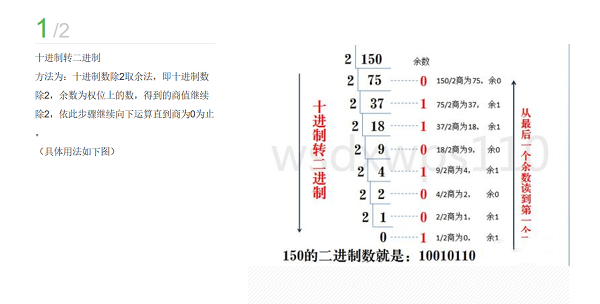# java中的运算符,ava运算符有哪些?

TheDisguiser 2020-07-18 20:31:00 java常见问答 4939

Java中运算符，一般分为四类：

1. 算数运算符：+ - * / % ++ --

”++“和”--“运算符，只允许用于数值类型的变量，不允许用于表达式中;"++"和”--“可以用于数值变量之前或者之后;

”++“和”--“用于数值变量之前，在赋值操作中，先对被”++“或”--“操作变量值先加1或者先减1，然后在进行其他的操作;

"++"和”--“用于数值变量之后，在赋值操作中，先用被”++“或”--“的操作变量值进行其他的操作，然后在对其值加1或者减1。

```package nums;
public class Test1
{
public static void main(String[] args)
{
int num1 = 20, num2 = 20;
System.out.println(++num1);
System.out.println(num2++);
}
}```
```package nums;
public class Test2
{
public static void main(String[] args)
{
int num1 = 10;
int num2 = 22;
double num3 = 52.2;
System.out.println("加：" + num1 + num2);
System.out.println("减：" + (num1 - num2));
System.out.println("乘：" + num2 * num3); //会提升精度
System.out.println("除：" + num3 / num1);
System.out.println("求余：" + num1 % num2);
}
}```

PS：算数运算符操作数必须是数值类型。

2. 关系运算符：== != > >= < <=

```package nums;
public class Test3
{
public static void main(String[] args)
{
System.out.println("9.5<8 :" + (9.5 < 8));
System.out.println("8.5<=8.5:" + (8.5 <= 8.5));
System.out.println("a~z：" + ((int)
'a') + "~" + ((int)
'z'));
System.out.println("A~Z：" + ((int)
'A') + "~" + ((int)
'Z'));
System.out.println("'A' < 'a':" + ('A' < 'a')); //字符'A'的Unicode编码值小于字符'a'
}
}```

3. 逻辑运算符：&& || ! ^ & |

PS：&和|运算是把逻辑表达式全部计算完，而&&和||运算具有短路计算功能。

4. 位运算符：& | ~ ^ >> << >>>Java运算符有哪些，其中逻辑运算符又有哪些?

java运算符优先级排序正确的是哪些?